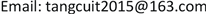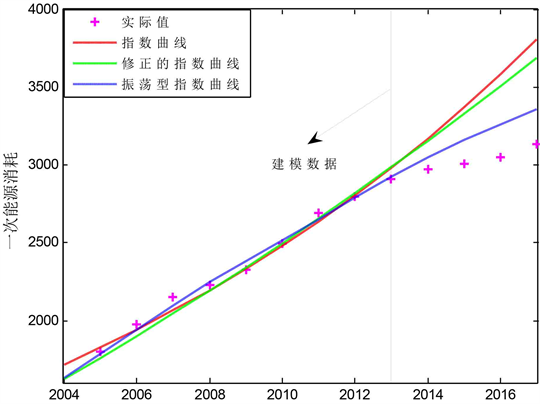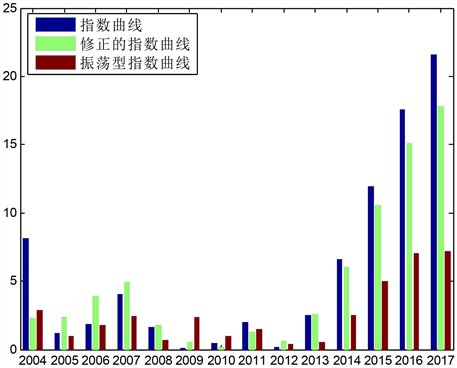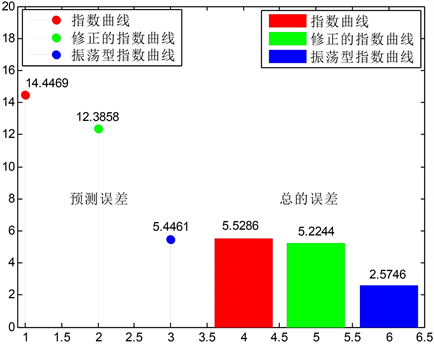﻿ 具有振荡项的指数曲线及其在一次能源消费中的应用 An Exponential Curve with Oscillating Term and Its Application in China’s Primary En-ergy Consumption

Operations Research and Fuzziology
Vol. 09  No. 02 ( 2019 ), Article ID: 30097 , 7 pages
10.12677/ORF.2019.92016

An Exponential Curve with Oscillating Term and Its Application in China’s Primary Energy Consumption

Shengyuan Tan

School of Computer Science, Chengdu University of Information Technology, Chengdu SichuanReceived: Apr. 16th, 2019; accepted: Apr. 30th, 2019; published: May 7th, 2019ABSTRACT

Aiming at modelling and prediction for oscillation sequences which exist widely in the real world, this paper proposes a new type of exponential curve model with oscillation term based on the classical exponential curve model. With the characteristics of the model and the least squares estimation method, an optimization problem is developed to evaluate the system parameters. Based on this problem, the Matlab software is used to solve the optimization problem to obtain the system parameters. Further, the model is applied to forecast the China’s primary energy consumption. The computational results are compared with the classical exponential curve and the modified exponential curve, and it shows the oscillating exponential curve has higher accuracy in China’s primary energy consumption.

Keywords:Exponential Curve, Oscillating, Least Squares Estimation, Primary Energy Consumption1. 引言

2. 指数曲线和修正的指数曲线

2.1. 指数曲线

${Y}_{t}=a{b}^{t}$(1)

$\mathrm{ln}{Y}_{t}=\mathrm{ln}a+t\mathrm{ln}b$(2)

$\left\{\begin{array}{l}\sum \mathrm{ln}Y=n\mathrm{ln}a+\left(\sum t\right)\mathrm{ln}b\\ \sum t\mathrm{ln}Y=\left(\sum t\right)\mathrm{ln}a+\left(\sum {t}^{2}\right)\mathrm{ln}b\end{array}$(3)

2.2. 修正指数曲线

${Y}_{t}=a{b}^{t}+K$(4)

${S}_{1}=\underset{t=0}{\overset{m-1}{\sum }}{Y}_{t}$${S}_{2}=\underset{t=m}{\overset{2m-1}{\sum }}{Y}_{t}$${S}_{2}=\underset{t=2m}{\overset{3m-1}{\sum }}{Y}_{t}$(5)

$\left\{\begin{array}{l}{S}_{1}=mK+a+ab+a{b}^{2}+\cdots +a{b}^{m-1}\\ {S}_{2}=mK+a{b}^{m}+a{b}^{m+1}+\cdots +a{b}^{2m-1}\\ {S}_{3}=mK+a{b}^{2m}+a{b}^{2m+1}+\cdots +a{b}^{3m-1}\end{array}$(6)

$\left\{\begin{array}{l}b={\left(\frac{{S}_{3}-{S}_{2}}{{S}_{2}-{S}_{1}}\right)}^{\frac{1}{m}}\\ a=\left({S}_{2}-{S}_{1}\right)\frac{b-1}{{\left({b}^{m}-1\right)}^{2}}\\ K=\frac{1}{m}\left({S}_{1}-\frac{a\left({b}^{m}-1\right)}{b-1}\right)\end{array}$(7)

3. 振荡型的指数曲线

${Y}_{t}=a{b}^{t}+ct+d+r\mathrm{sin}\left(t\right)$(8)

$\left\{\begin{array}{c}{Y}_{1}=\stackrel{^}{a}\stackrel{^}{b}+\stackrel{^}{c}+\stackrel{^}{d}+\stackrel{^}{r}\mathrm{sin}\left(1\right),\text{\hspace{0.17em}}\text{ }\\ {Y}_{2}=\stackrel{^}{a}{\stackrel{^}{b}}^{2}+2\stackrel{^}{c}+\stackrel{^}{d}+\stackrel{^}{r}\mathrm{sin}\left(2\right),\text{\hspace{0.17em}}\\ ⋮\\ {Y}_{m}=\stackrel{^}{a}{\stackrel{^}{b}}^{m}+m\stackrel{^}{c}+\stackrel{^}{d}+\stackrel{^}{r}\mathrm{sin}\left(m\right).\end{array}$ (9)

$S=\underset{a,b,c,d,r}{\mathrm{min}}\underset{i=1}{\overset{m}{\sum }}{\left\{x\left(1\right)-\left(a{b}^{i}+ci+d+r\mathrm{sin}\left(i\right)\right)\right\}}^{2}$(10)

$\left\{\begin{array}{c}\frac{\partial S}{\partial a}=\underset{i=1}{\overset{m}{\sum }}\left\{x\left(1\right)-\left(a{b}^{i}+ci+d+r\mathrm{sin}\left(i\right)\right)\right\}{b}^{i}=0,\text{ }\text{\hspace{0.17em}}\\ \frac{\partial S}{\partial b}=\underset{i=1}{\overset{m}{\sum }}\left\{x\left(1\right)-\left(a{b}^{i}+ci+d+r\mathrm{sin}\left(i\right)\right)\right\}ai{b}^{i-1}=0,\\ \frac{\partial S}{\partial c}=\underset{i=1}{\overset{m}{\sum }}\left\{x\left(1\right)-\left(a{b}^{i}+ci+d+r\mathrm{sin}\left(i\right)\right)\right\}i=0,\text{ }\text{ }\\ \begin{array}{l}\frac{\partial S}{\partial d}=\underset{i=1}{\overset{m}{\sum }}\left\{x\left(1\right)-\left(a{b}^{i}+ci+d+r\mathrm{sin}\left(i\right)\right)\right\}=0,\\ \frac{\partial S}{\partial r}=\underset{i=1}{\overset{m}{\sum }}\left\{x\left(1\right)-\left(a{b}^{i}+ci+d+r\mathrm{sin}\left(i\right)\right)\right\}\mathrm{sin}\left(i\right)=0.\end{array}\end{array}$ (11)

4. 应用实例Table 1. The statistical data of the primary energy consumption of China (mtoe)

$\text{APE}=|\frac{{Y}_{t}-{\stackrel{^}{Y}}_{t}}{{Y}_{t}}|×100%,t=1,2,\cdots ,n$(12)

$\text{MAPE}=\frac{1}{v-l+1}\underset{t=l}{\overset{v}{\sum }}|\frac{{Y}_{t}-{\stackrel{^}{Y}}_{t}}{{Y}_{t}}|×100%,v\le n$(13)Figure 1. Modelling and forecasting the primary energy consumption by the exponential curve, the modified exponential curve and the oscillation exponential curve modelsTable 2. The results of China’s primary energy consumption by the exponential curve, the modified exponential curve and the oscillation exponential curve modelsFigure 2. The absolute percentage errors of the primary energy consumption by the exponential curve, the modified exponential curve and the oscillation exponential curve modelsFigure 3. The forecasting error and the total error of the primary energy consumption by the exponential curve, the modified exponential curve and the oscillation exponential curve models

5. 结论

An Exponential Curve with Oscillating Term and Its Application in China’s Primary En-ergy Consumption[J]. 运筹与模糊学, 2019, 09(02): 140-146. https://doi.org/10.12677/ORF.2019.92016

1. 1. BP (2018) BP Statistical Review of World Energy 2018.

2. 2. 宋廷山, 王坚, 刁艳华, 郭思亮. 应用统计学——以EXCEL为分析工具[M]. 第2版. 北京: 清华大学出版社, 2018.

3. 3. 秦尚林, 陈善雄, 许锡昌. 路基沉降预测的拓展指数曲线模型[J]. 铁道标准设计, 2010(2): 28-30.

4. 4. 刘芳, 潘毅, 刘辉, 丁强, 李强, 王芝茗. 风电功率预测误差分段指数分布模型[J]. 电力系统自动化, 2013, 37(18): 14-19.

5. 5. 杨桂元. 指数曲线预测模型的参数估计与误差分析[J]. 运筹与管理, 2003, 12(4): 55-58.

6. 6. 李晓凤. 修正指数曲线的人口预测模型[J]. 荆门职业技术学院学报, 2006, 21(6): 85-88.

7. 7. 王吉权. 修正指数曲线在电力负荷预测中的应用[J]. 工程应用技术与实现, 2006, 32(18): 253-254, 277.

8. 8. 欧阳明, 丁伯阳, 石吉森. 单桩荷载–沉降曲线的修正指数曲线拟合研究[J]. 水运工程, 2013(1): 31-38.

9. 9. 石文杰. 修正指数曲线法在软土路基沉降预测中的实践[J]. 中国标准化, 2017(8): 164-168.

10. 10. 王正新. 振荡型GM(1,1)幂模型及其应用[J]. 控制与决策, 2013, 28(10): 1459-1472.